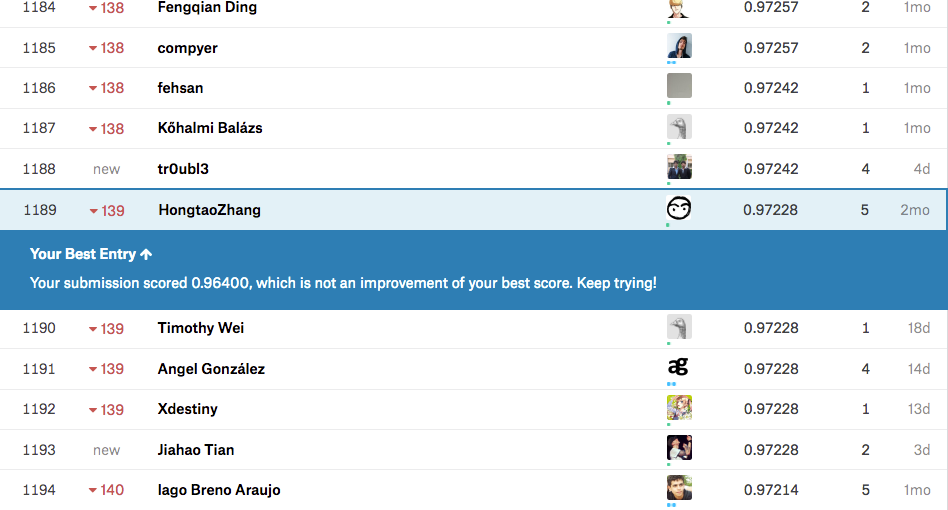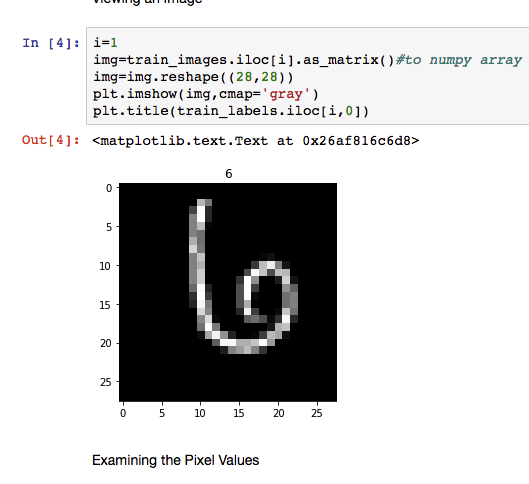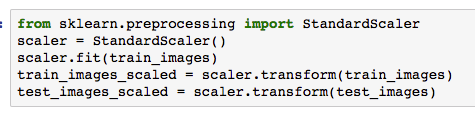0
0
01. 云栖社区>
2. 博客>
3. 正文

机器学习实战篇——用支撑向量算法在Kaggle上跑个分

hongtao2018 2018-06-18 17:25:00 浏览404一、下载处理数据

import pandas as pd
import matplotlib.pyplot as plt, matplotlib.image as mpimg
from sklearn.model_selection import train_test_split
from sklearn import svm
%matplotlib inline

images = labeled_images.iloc[:,1:]
labels = labeled_images.iloc[:,:1]
train_images, test_images,train_labels, test_labels = train_test_split(images, labels,
train_size=0.95, random_state=0)

train_test_split 函数是用来将数据成两组，训练组和验证组，其中训练组占95%。image.pngimage.png

二、用Sklearn的SVM学习数据

from sklearn.svm import SVC
clf = svm.SVC(kernel = "poly", degree = 3, coef0=0.1, C=100)
clf.fit(train_images_scaled, train_labels.values.ravel())
clf.score(test_images_scaled,test_labels)

三、用训练好的分类器来标记数据

test_data_scaled = scaler.transform(test_data)
results=clf.predict(test_data_scaled)

————

AI学习笔记——循环神经网络（RNN）的基本概念
AI学习笔记——神经网络和深度学习
AI学习笔记——卷积神经网络1（CNN）
————

hongtao2018
+ 关注